• 吴清强老师的作业，但感觉网上的答案都有些许错误。
#include<iostream>
#include<fstream>
#include<queue>
using namespace std;

struct A
{
int arrive;		//到达时间
int time;		//服务时间
int start;		//开始时间
int end;		//结束时间
float rate;		//响应比
char name;		//进程名称
bool activated; //是否到达
};

void FCFS(A a[],int n)
{
cout<<"先到先服务："<<endl;
for(int i=0;i<n;i++)
{
if(i!=0)
{
if(a[i].arrive<a[i-1].end)
{
a[i].start = a[i-1].end;
a[i].end = a[i].start+a[i].time;
}
}
else
{
a[i].start = a[i].arrive;
a[i].end = a[i].start+a[i].time;
}
a[i].rate = (float)(a[i].end-a[i].arrive)/a[i].time;
cout<<a[i].name<<"的完成时间:  "<<a[i].end<<"   周转时间:  "<<a[i].end-a[i].arrive<<"   响应比:  "<<a[i].rate<<endl;
}

}

void RR(A a[],int n)
{
queue <A> q;
A temp;
cout<<"RR(q=1):"<<endl;
A b;
for(int i=0;i<n;i++)
{
b[i] = a[i];
}
int i=0,j=1;
q.push(a);
while(!q.empty())
{
temp = q.front();
cout<<temp.name<<endl;
q.pop();
temp.time--;
if(temp.time != 0)
{
q.push(temp);
}
else
{
temp.end = j;
for(int i=0;i<n;i++)
{
if(temp.name == a[i].name)
{
a[i] = temp;
cout<<a[i].end<<" "<<a[i].arrive<<" "<<a[i].time<<endl;;
a[i].rate = (float)(a[i].end-a[i].arrive)/b[i].time;
}
}
}
for(int i=1;i<n;i++)
{
if(a[i].arrive == j)
{
q.push(a[i]);
}
}
j++;
}
for(int i=0;i<n;i++)
{
cout<<a[i].name<<"的完成时间:  "<<a[i].end<<"   周转时间:  "<<a[i].end-a[i].arrive<<"   响应比:  "<<a[i].rate<<endl;
}
}

//最短优先
void SPN(A a[],int n)
{
cout<<"SPN:"<<endl;
int i=0,j=0,now=0,min=100;
A b;
//将a拷贝给b
for(int i=0;i<n;i++)
{
b[i] = a[i];
}
while(i!=n-1)
{
//检查所有进程，是否已到达
for(int k=0;k<n;k++)
{
//将到达的进程放入排序队列
if(b[k].arrive == j)
{
b[k].activated = true;
a[k].activated = true;
}
}

//循环遍历找到当前既在排序队列中又最短的进程
for(int l=0;l<n;l++)
{
if(a[l].time<min && a[l].activated==true)
{
min = a[l].time;
now = l;
}
}
min = 100;
b[now].time--;
if(b[now].time == 0)
{
i++;
b[now].activated = false;
a[now].activated = false;
a[now].end = j+1;
a[now].rate = (float)(a[now].end-a[now].arrive)/a[now].time;
}
j++;
}
//输出
for(int i=0;i<n;i++)
{
cout<<a[i].name<<"的完成时间:  "<<a[i].end<<"   周转时间:  "<<a[i].end-a[i].arrive<<"   响应比:  "<<a[i].rate<<endl;
}
}
//最短剩余
void SRT(A a[],int n)
{
cout<<"SRT:"<<endl;
int i=0,j=0,now=0,min=100;
A b;
//将a拷贝给b
for(int i=0;i<n;i++)
{
b[i] = a[i];
}
while(i!=n-1)
{
//检查所有进程，是否已到达
for(int k=0;k<n;k++)
{
//将到达的进程放入排序队列
if(b[k].arrive == j)
{
b[k].activated = true;
}
}

//循环遍历找到当前既在排序队列中又最短的进程
for(int l=0;l<n;l++)
{
if(b[l].time<min && b[l].activated==true)
{
min = a[l].time;
now = l;
}
}
min = 100;
b[now].time--;
if(b[now].time == 0)
{
i++;
b[now].activated = false;
a[now].end = j+1;
a[now].rate = (float)(a[now].end-a[now].arrive)/a[now].time;
}
j++;
}
//输出
for(int i=0;i<n;i++)
{
cout<<a[i].name<<"的完成时间:  "<<a[i].end<<"   周转时间:  "<<a[i].end-a[i].arrive<<"   响应比:  "<<a[i].rate<<endl;
}
}

void HRRN(A a[],int n)
{
cout<<"HRRN:"<<endl;
int i=0,j=0,now=0,min=100,rate = 0;
A b;
//将a拷贝给b
for(int i=0;i<n;i++)
{
b[i] = a[i];
}
while(i!=n-1)
{
//检查所有进程，是否已到达
for(int k=0;k<n;k++)
{
//将到达的进程放入排序队列
if(b[k].arrive == j)
{
b[k].activated = true;
a[k].activated = true;
}
}

for(int l=0;l<n;l++)
{
if(b[l].activated == true && b[l].rate>rate)
{
rate = b[l].rate;
now = l;
}
}
rate = 0;
b[now].rate = (float)(j+1-a[now].arrive)/a[now].time;
b[now].time--;
if(b[now].time == 0)
{
i++;
b[now].activated = false;
a[now].end = j+1;
a[now].rate = (float)(a[now].end-a[now].arrive)/a[now].time;
}
j++;
}
//输出
for(int i=0;i<n;i++)
{
cout<<a[i].name<<"的完成时间:  "<<a[i].end<<"   周转时间:  "<<a[i].end-a[i].arrive<<"   响应比:  "<<a[i].rate<<endl;
}
}

int main()
{
A a;

ifstream in("data.txt");
//输入
for(int i=0;i<5;i++)
{
in>>a[i].name;
in>>a[i].arrive;
in>>a[i].time;
a[i].activated = false;
a[i].rate = 1;
}
in.close();

FCFS(a,5);
RR(a,5);
SRT(a,5);
SPN(a,5);
HRRN(a,5);
system("pause");
return 0;
}
展开全文• 实验处理器调度实验内容 选择一个调度算法实现处理器调度实验目的 在采用多道程序设计的系统中 往往有若干个进程同时处于就绪状态 当就绪状态进程 个数大于处理器数时 就必须依照某种策略来决定哪些进程...
• 实验处理器调度 一. 实验内容 选择一个调度算法，实现处理器调度。 二. 实验目的 在采用多道程序设计的系统中，往往有若干个进程同时处于就绪状态。当就绪状态进程个数大于处理器数时，就必须依照某种策略来决定...
• 实验目的与要求 ...本实验模拟在单处理器情况下处理器调度，帮助学生加深了解处理器调度的工作。 二、实验要求   （1） 实验题目。 （2） 程序中使用的数据结构及符号说明。 ...

实验目的与要求

一、实验目的

在采用多道程序设计的系统中，往往有若干个进程同时处于就绪状态。当就绪状态进程个数大于处理器数时，就必须依照某种策略来决定哪些进程优先占用处理器。本实验模拟在单处理器情况下处理器调度，帮助学生加深了解处理器调度的工作。

二、实验要求

（1）   实验题目。

（2）   程序中使用的数据结构及符号说明。

（3）   流程图。

（4）   打印一份源程序并附上注释。

（5）   打印程序运行时的初值和运行结果，要求如下：

1 进程控制块的初始状态

2.选中运行的进程名以及选中进程运行后的各进程控制块状态。

对于2要求每选中一个进程运行后都要打印。

实验原理与内容

三、实验原理

假定系统有五个进程，每一个进程用一个进程控制块PCB来代表。进程控制块的格式为：

进程名

时间

要求求运行时间

优先数

状态

其中，进程名----作为进程的标识，假设五个进程的进程名分别是P1，P2，P3，P4，P5。

指针----按优先数的大小把五个进程连成队列，用指针指出下一个进程的进程控制块

首地址，最后一个进程中的指针为“0”。

要求运行时间----假设进程需要运行的单位时间数。

优先数----赋予进程的优先数，调度时总是选取优先数大的进程先执行。

状态----可假设有两种状态，“就绪”状态和“结束“状态，五个进程的初始状态都为“就绪“状态，用“R”表示，当一个进程运行结束后，它的状态变为“结束”，

用“E”表示。

在每次运行你所设计的处理器调度程序之前，为每个进程任意确定它的“优先数”和“要求运行时间”。

为了调度方便，把五个进程按给定的优先数从大到小连成队列，用一单元指出队首进程，用指针指出队列的连接情况。例：

队首标志

k2

k1                  k2                 k3                 k4               k5

P1

0

2

1

P3

k5

1

3

P5

k1

4

2

P4

k3

2

4

P2

k4

3

5

R

R

R

R

R

PCB1            PCB2          PCB3           PCB4          PCB5

处理器调度总是选队首进程运行。采用动态改变优先数的办法，进程每运行一次优先数就减“1”。由于本实验是模拟处理器调度，所以，对被选中的进程并不实际的启动运行，而是执行：

优先数－1

要求运行时间－1

来模拟进程的一次运行。

提醒注意的是：在实际的系统中，当一个进程被选中运行时，必须恢复进程的现场，它占有处理器运行，直到出现等待事件或运行结束。在这里省去了这些工作。

进程运行一次后，若要求运行时间≠0，则再将它加入队列（按优先数大小插入，且置队首标志）；若要求运行时间=0，则把它的状态修改为“结束”（），且退出队列。

若“就绪”状态的进程队列不为空，则重复上面（4）和（5）的步骤，直到所有进程都成为“结束”状态。

在所设计的称序中应有显示或打印语句，能显示或打印每次被选中进程的进程名以及运行一次后进称对列的变化。

为五个进程任意确定一组“优先数”和“要求运行时间”，启动所设计的处理器调度程序，显示或打印逐次被选中进程的进程名以及进程控制块的动态变化过程。

实验过程与结果（可贴图）

一．实验题目

设计一个按优先数调度算法实现处理器调度的进程。

//定义结构体，包含五个属性，名字，下一个PCB指针，运行时间，优先级，目前状态

（一）  程序中使用的数据结构及符号说明。

本程序采用的进程结构体为PCB

struct PCB /*进程控制块结构体,包含标识符、优先数、运行时间、状态、前后指针*/
{

charname;  /*进程标识符*/

intrun_time;  /*进程运行时间*/

intprior_num;  /*进程优先数*/

charstatus;  /*进程状态：R-就绪,E-结束*/

structPCB *pre;  /*指向后一进程的指针*/

structPCB *next;  /*指向后一进程的指针*/

};

/*数据结构为双链表*/

struct PCB *tail;  /*进程链表的尾指针*/

（四）源程序

#include<stdio.h>

#include<stdlib.h>

#include<time.h>

int num = 5;  /*假定进程数为5*/

struct PCB*tail;  /*进程链表的尾指针*/

intglobal_time;  /*定义全局时间*/

struct PCB*PCBinit(struct PCB *q);   /*初始化进程链表*/

struct PCB*init(struct PCB *p, int i);  /*初始化进程*/

voidexchange(struct PCB *p, struct PCB *max); /*交换相邻两个进程的指针*/

void run(structPCB *p);  /*模拟运行进程*/

voidcheck_runtime(struct PCB *p);/*判断运行时间是否为0*/

struct PCB  /*进程控制块结构体,包含标识符、优先数、运行时间、状态、前后指针*/

{

char name;  /*进程标识符*/

int run_time;  /*进程运行时间*/

int prior_num;  /*进程优先数*/

char status;  /*进程状态：R-就绪,E-结束*/

struct PCB *pre;  /*指向后一进程的指针*/

struct PCB *next;  /*指向后一进程的指针*/

};

//初始化进程

struct PCB*init(struct PCB *p, int i){

//初始化进程名

p->name = 'P';

p->name = 'C';

p->name = 'B';

p->name = i+1+'0';

//为进程指定运行时间

printf("进程 %s\n",p->name);

printf("请确定该进程的运行时间：");

scanf("%d", &p->run_time);

//为进程指定优先数

printf("请确定该进程的优先数：");

scanf("%d",&p->prior_num);

printf("\n");




//初始化进程状态为就绪

p->status = 'R';

//初始化指向后一进程的指针为空

p->next = NULL;

//返回进程

return p;

}

//初始化进程链表

struct PCB*PCBinit(struct PCB *q){

int i;

struct PCB *p = NULL;  /*p为待运行队列PCB指针*/

head = tail = NULL; /*初始化头尾指针*/

for(i = 0; i < num; i++){

p = (struct PCB*)malloc(sizeof(struct PCB));  //分配空间，让p指向这个PCB

init(p,i); //初始化进程

p->next = NULL;

p->pre = NULL;

}else{

p->pre = tail;

tail->next = p;

tail = p;

}

}

return p;

}

//冒泡排序链表

struct PCB *a, *b;  //定义进程a,b

int i;

for(i=0;i<num;i++){  //外循环循环次数取决于全局变量num，相当于链表长度

while(b != NULL){  //b非空

if(a->prior_num <b->prior_num){  //比较进程a,b的优先数

exchange(a,b);  //调用exchange函数交换a,b进程指针

a = a->pre;  //实现一次指针交换要重置a,b进程位置

b = b->next;  //因为指针交换数据不变

}

a = a->next; //完成一次内循环后

b = b->next; //指针顺延

}

}

}

//交换相邻两个进程的指针

void exchange(structPCB *p, struct PCB *max){

if(p == max | max != p->next){  //判断若两个进程不相邻，则返回

return;

}

if(max == p->next){  //进程相邻

if(p->pre != NULL){  //进程p指向前一进程的指针不为空

p->pre->next = max;  //将p的前一进程的后指针指向max

}

if(max->next != NULL){  //max的下一进程指针不为空

max->next->pre=p;  //将max的下一进程的前指针指向p

}

max->pre = p->pre;  //将max的前一指针指向p的前一指针

p->next = max->next;  //将p的后一指针指向max的后一指针

max->next = p;  //max的后一指针指向p

p->pre = max;  //p的前一指针指向max

}//本方法用于实现冒泡排序中进程结构体只转换指针，不转换数据

}

//输出进程信息

struct PCB *p;

for(p = phead; p != NULL; p =p->next){

printf("进程 %s\t 优先数 %d\t 运行时间 %d\t 状态 %c\n",p->name,p->prior_num,p->run_time,p->status);

}

}

//运行函数

void run(structPCB *p){

//输出这个任务的状态，全局时间加1，任务还需要的时间减1，优先级减1

global_time++;  //全局时间加1

p->run_time--;  //运行时间减1

if(p->prior_num > 0)   // 优先数需大于0

p->prior_num--;   //优先数减1

printf("第 %d 次运行:\n",global_time);

printf("当前进程: %s\t 优先数 %d\t 运行时间:%d\n", p->name, p->prior_num, p->run_time);

printf("\n");

}

//判断运行时间是否为0

voidcheck_runtime(struct PCB *p){

if(p->run_time <= 0){  //当运行时间为0，结束进程

p->status = 'E';  //修改进程状态

printf("进程 %s 已结束",p->name);

printf("\n");

printf("进程当前状态为：\n");

printf("\n");

printf("-----------------------------------------------------\n");

printf("请按回车键进行下一进程");

printf("\n");

getchar();  //接收回车键

free(p); //释放p内存

}

}

void main(){

struct PCB *p = NULL;  /*p为待运行队列PCB指针*/

//初始化进程链表

PCBinit(p);

//按优先数递减进行进程链表排序

printf("进程当前状态为：\n");

printf("\n");

//若链表中还有PCB是执行循环语句

run(p);   //进行调度

check_runtime(p); //判断运行时间是否为0

}

}

（五）程序运行时的初值和运行结果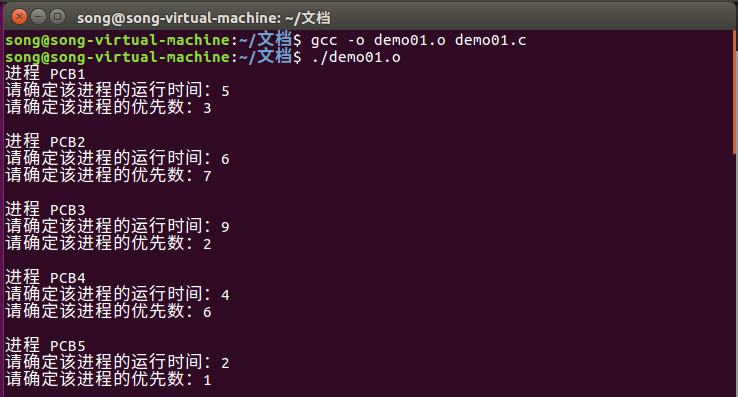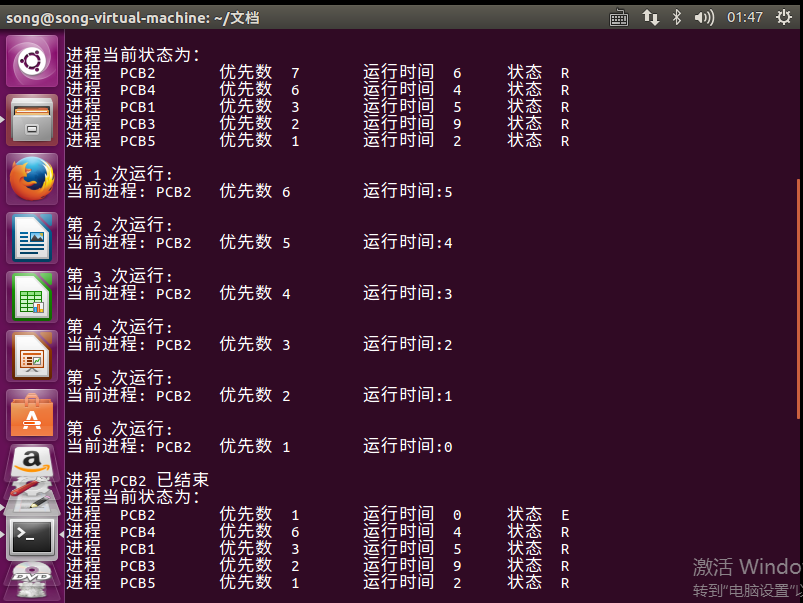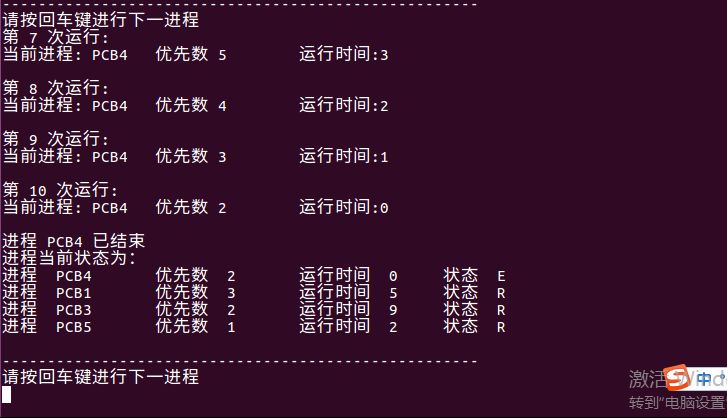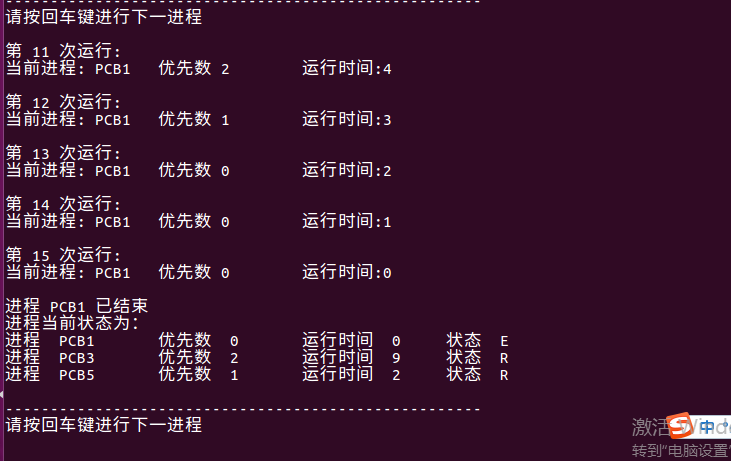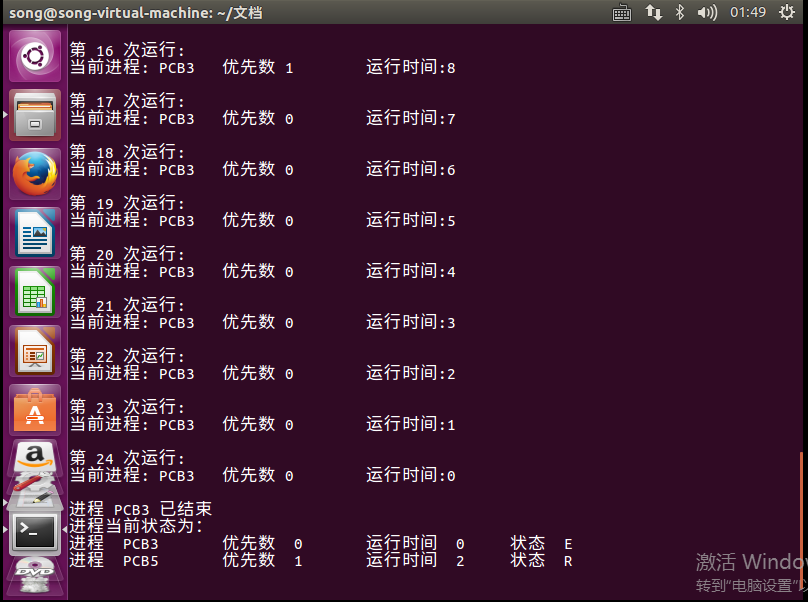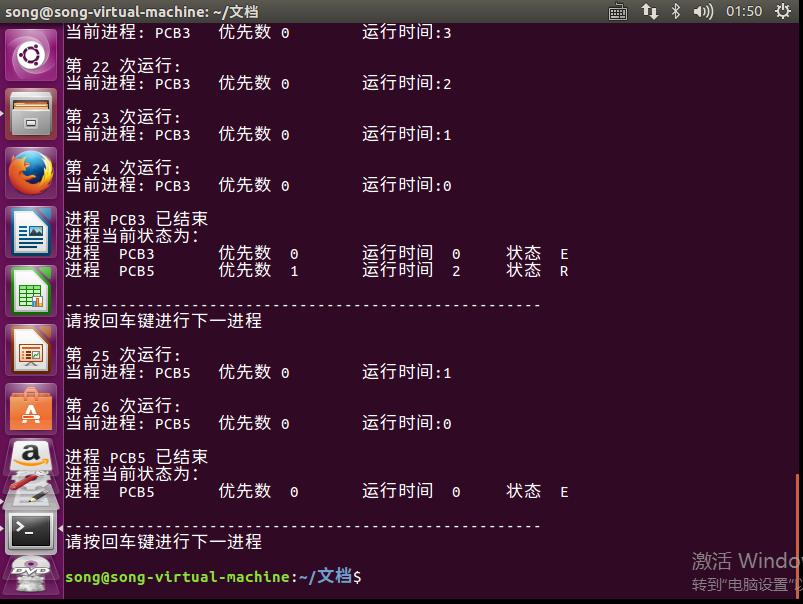展开全文• 在采用多道程序设计的系统中，往往有若干个进程同时处于就绪状态。当就绪进程个数大于处理器数时，就必须依照某种策略来决定哪些进程优先...本实验模拟在单处理器情况下的处理器调度，帮助学生加深了解处理器调度的工作
• 随机给出一个进程调度实例，如： 进程 到达时间 服务时间 A 0 3 B 2 6 C 4 4 D 6 5 E 8 2 模拟进程调度，给出按照算法先来先服务FCFS、轮转RR（q=1）、最短进程优先SPN、最短...实验报告（含流程图及运行结果）&源码
• #include #include<queue>//调用STL中的优先队列 using namespace std; //定义一个PCB进程类 class PCB { public: char name;//进程的名字 int runtime;//该进程的运行时间 int priority;...……
• 实验处理器调度 一. 实验内容 选择一个调度算法，实现处理器调度。 二. 实验目的 在采用多道程序设计的系统中，往往有若干个进程同时处于就绪状态。当就绪状态进程个数大于处理器数时，就必须依照某种策略来...
• 进程调度实验 一、实验目的 多道系统中，当就绪进程数大于处理机数时，必须按照某种策略决定选取哪些进程占用处理器。本实验模拟实现处理器调度，进一步加深对处理器调度算法的理解。 1、设计一个有N个进程并发的...操作系统
• 实验处理器调度 一实习内容 选择一个调度算法实现处理器调度 二实习目的 在采用多道程序设计的系统中 往往有若干个进程同时处于就绪状态 当就绪进程个数 大于处理器数时 就必须依照某种策略来决定哪些进程优先...
• PAGE PAGE # 实验处理器调度实验内容 选择一个调度算法实现处理器调度实验目的 在采用多道程序设计的系统中 往往有若干个进程同时处于就绪状态 当就绪进程个数 大于处理器数时就必须依照某种策略来决定...
• 处理器调度实验——FCFS, RR, SPN, SRT, HRRN实验题目代码 实验题目 随机给出一个进程调度实例，如： 进程 到达时间 服务时间 A 0 3 B 2 6 C 4 4 D 6 5 E 8 2 模拟进程调度，给出按照算法先来先服务FCFS、...
处理器调度实验——FCFS, RR, SPN, SRT, HRRN实验题目代码
实验题目
随机给出一个进程调度实例，如：
进程   到达时间  服务时间
A       0			3
B       2			6
C       4			4
D       6			5
E       8			2
模拟进程调度，给出按照算法先来先服务FCFS、轮转RR（q=1）、最短进程优先SPN、最短剩余时间SRT、最高响应比优先HRRN进行调度各进程的完成时间、周转时间、响应比的值。
代码
#include <iostream>
#include <queue>
using namespace std;

struct A
{
char name;      //进程名称
int arrive;     //到达时间
int time;       //服务时间
int start;      //开始时间
int end;        //结束时间
float rate;     //响应比
bool activated; //是否到达
int rest;       //剩余时间
};

/*
结束时间=开始时间+服务/执行时间(无抢断)
周转周期=结束时间-到达时间
响应比的值(带权周转时间)=周转时间/服务时间
=(结束时间-到达时间)/服务时间
*/

void FCFS(A a[], int n)
{
//先来先服务是最简单的策略，也成为先进先出FIFO。首先它是一个非抢占的。
//如字面的意思，它根据进程到达时间决定先运行哪一个进程。

for (int i = 0; i < n; i++)
{
if (i > 0 && a[i].arrive < a[i - 1].end)
{
a[i].start = a[i - 1].end;
a[i].end = a[i].start + a[i].time;
}
else
{
a[i].start = a[i].arrive;
a[i].end = a[i].start + a[i].time;
}

a[i].rate = (float)(a[i].end - a[i].arrive) / a[i].time; //求相应比
cout << a[i].name << " " << a[i].end << " " << a[i].end - a[i].arrive << " " << a[i].rate << endl;
//进程名 进程结束时间 周转周期 响应比
}
}

void RR(A a[], int n)
{
//轮转也称时间片技术（time slicing，SL），对于轮转法，最重要的是时间片的长度
//转算法以一个周期（q）产生中断，当中断发生时，当前运行的程序置于就绪队列（队尾）
//中，然后基于FCFS选择下一个就绪作业运行
queue<A> q;//轮转队列
A temp;

for (int i = 0; i < n; i++)
{
b[i] = a[i];
}

int time_now = 0;
q.push(a);
while (!q.empty())
{
time_now++;
temp = q.front();
q.pop();//删除队首元素
temp.time--;

for (int i = 1; i < n; i++)
{
if (a[i].arrive == time_now)//就绪进程进入队尾
{
q.push(a[i]);
}
}
if (temp.time != 0)//当前进程未运行完
{
q.push(temp);//把当前运行进程插入队尾
}
else
{
temp.end = time_now;
for (int i = 0; i < n; i++)
{
if (temp.name == a[i].name)
{
a[i] = temp;
a[i].rate = (float)(a[i].end - a[i].arrive) / b[i].time;
}
}
}
}
for (int i = 0; i < n; i++)
{
cout << a[i].name << " " << a[i].end << " " << a[i].end - a[i].arrive << " " << a[i].rate << endl;
}
}

void SPN(A a[], int n) //最短进程优先SPN
{
//最短作业优先（Short Job First,SJF）。
//它也是一个非抢占的。是根据服务的时间经行选择。
//要注意下到达时间的顺序，有多个进程等待时选择服务时间最少的进程

int now = 0;                //正在运行进程标志
int min = 100;              //最短进程时间
int time_now = a.arrive; //标志当前时间
//假设输入默认按到达时间升序或默认a最早到达

for (int i = 0; i < n; i++)
{
for (int k = 0; k < n; k++) //检查所有进程，是否已到达
{
if (a[k].arrive <= time_now && a[k].time != 0) //获取到达的进程
{
a[k].activated = true;
}
}

for (int l = 0; l < n; l++) //循环遍历找到当前既到达又最短的进程 a[now]
{
if (a[l].time < min && a[l].activated == true)
{
min = a[l].time;
now = l;
}
}

a[now].start = time_now;
a[now].end = a[now].start + a[now].time; //当前进程结束时间
time_now = a[now].end;                   //下一个进程开始时间
a[now].rate = (float)(a[now].end - a[now].arrive) / a[now].time;
a[now].activated = false;
a[now].time = 0; //去除当前进程
min = 100;
}

for (int i = 0; i < n; i++) //输出
{
cout << a[i].name << " " << a[i].end << " " << a[i].end - a[i].arrive << " " << a[i].rate << endl;
}
}

void SRT(A a[], int n) //最短剩余时间优先SRT
{
//SRT是针对SPN增加了抢占机制的版本，
//就好比某个进程运行时间非常长，在这期间其他所有的进程都在等待，
//如果将其中断，先处理所需时间少的，运行效率会有显著提升。
int now = 0;                //正在运行进程标志
int min = 100;              //最短进程时间
int time_now = a.arrive; //标志当前时间
//假设输入默认按到达时间升序

for (int i = 0; i < n; i++)
a[i].rest = a[i].time;
for (int i = 0; i < n; i++)
{
for (int j = 0;; j++)
{
for (int k = 0; k < n; k++) //检查所有进程，是否已到达
{
if (a[k].arrive <= time_now && a[k].time != 0) //获取到达的进程
{
a[k].activated = true;
}
}

for (int l = 0; l < n; l++)
{
if (a[l].rest < min && a[l].activated == true)//找到当前既到达剩余又最短的进程 a[now]
{
min = a[l].rest;
now = l;
}
}
a[now].start = time_now;
int m = 0;
for (m = 0; m < n; m++)
{
if (m != now && a[m].arrive < time_now + a[now].rest && a[m].time != 0 && a[m].activated == false)
{//找到当前到达的进程
time_now = a[m].arrive;
a[now].rest = a[now].start + a[now].rest - time_now;
if (a[m].rest < a[now].rest)//抢断
{
now = m;
break;
}
}
}
if (m == n)//当前进程未被抢断，运行完成
{
a[now].end = time_now + a[now].rest;
time_now = a[now].end; //下一个进程开始时间
a[now].rate = (float)(a[now].end - a[now].arrive) / a[now].time;
a[now].activated = false;
a[now].time = 0; //去除当前进程
break;
}
}
min = 100;
}

for (int i = 0; i < n; i++) //输出
{
cout << a[i].name << " " << a[i].end << " " << a[i].end - a[i].arrive << " " << a[i].rate << endl;
}
}

void HRRN(A a[], int n) //高响应比优先HRRN
{
//高响应比优先调度算法主要用于作业调度，
//该算法是对FCFS调度算法和SJF调度算法的一种综合平衡，
//同时考虑每个作业的等待时间和估计的运行时间。在每次进行作业调度时，
//先计算后备作业队列中每个作业的响应比，从中选出响应比最高的作业投入运行
//A 3 3 1
//B 9 7 1.16667
//C 13 9 2.25
//D 20 14 2.8
//E 15 7 3.5

int now = 0;                //正在运行进程标志
int min = 1;                //最短进程时间
int time_now = a.arrive; //标志当前时间
//假设输入默认按到达时间升序或默认a最早到达

for (int i = 0; i < n; i++)
{
for (int k = 0; k < n; k++) //检查所有进程，是否已到达
{
if (a[k].arrive <= time_now && a[k].time != 0) //获取到达的进程
{
a[k].activated = true;
}
}

for (int l = 0; l < n; l++) //循环遍历找到当前既到达又响应比最高 a[now]
{

if (a[l].activated == true && a[l].time != 0)
{
a[l].rate = (float)(time_now + a[l].time - a[l].arrive) / a[l].time;
//计算当前响应比

if (min < a[l].rate)//找出相应比高的进程
{
min = a[l].rate;
now = l;
}
}
}

a[now].start = time_now;
a[now].end = a[now].start + a[now].time; //当前进程结束时间
time_now = a[now].end;                   //下一个进程开始时间
a[now].time = 0; //去除当前进程
min = 1;
}

for (int i = 0; i < n; i++) //输出
{
cout << a[i].name << " " << a[i].end << " " << a[i].end - a[i].arrive << " " << a[i].rate << endl;
}
}
int main()
{
int num;
cin >> num;

//输入
for (int i = 0; i < num; i++)
{
cin >> a[i].name;
cin >> a[i].arrive;
cin >> a[i].time;
a[i].activated = false;
a[i].rate = 1;
}
string method;
cin >> method; //获取算法名称

/*请在以下区域填入代码*/
if (method == "FCFS")
{
FCFS(a, num);
}
if (method == "RR")
{
RR(a, num);
}
if (method == "SPN")
{
SPN(a, num);
}
if (method == "SRT")
{
SRT(a, num);
}
if (method == "HRRN")
{
HRRN(a, num);
}

system("pause");
return 0;
}



展开全文• 在多道程序或多任务系统中，系统中同时处于就绪态的进程有若干个，也就是说能运行的进程数远远大于处理器个数。为了使系统中的各进程能...本实验要求设计一个模拟单处理器调度的算法，以加深对处理器调度的概念理解。
• 实验处理器调度   一、实验内容 按优先数调度算法实现处理器调度。 二、实验目的 在采用多道程序设计的系统中，往往有若干个进程同时处于就绪状态。当就绪进程个数大于处理器数时，就必须依照某种策略来...
实验1   处理器调度

一、实验内容

按优先数调度算法实现处理器调度。

二、实验目的

在采用多道程序设计的系统中，往往有若干个进程同时处于就绪状态。当就绪进程个数大于处理器数时，就必须依照某种策略来决定哪些进程优先占用处理器。本实验模拟在单处理器情况下的处理器调度，帮助学生加深了解处理器调度的工作。

三、实验原理

设计一个按优先数调度算法实现处理器调度的程序。

(1) 假定系统有五个进程，每一个进程用一个进程控制块PCB来代表，进程控制块的格式为：

进程名

指针

要求运行时间

优先数

状态

其中，进程名——作为进程的标识，假设五个进程的进程名分别为P1，P2，P3，P4，P5。

指针——按优先数的大小把五个进程连成队列，用指针指出下一个进程的进程控制块的首地址，最后一个进程中的指针为“0”。

要求运行时间——假设进程需要运行的单位时间数。

优先数——赋予进程的优先数，调度时总是选取优先数大的进程先执行。

状态——可假设有两种状态，“就绪”状态和“结束”状态。五个进程的初始状态都为“就绪”，用“R”表示，当一个进程运行结束后，它的状态为“结束”，用“E”表示。

(2) 在每次运行你所设计的处理器调度程序之前，为每个进程任意确定它的“优先数”和“要求运行时间”。

(3) 为了调度方便，把五个进程按给定的优先数从大到小连成队列。用一单元指出队首进程，用指针指出队列的连接情况。例：

队首标志

K2

K1

P1

K2

P2

K3

P3

K4

P4

K5

P5

0

K4

K5

K3

K1

2

3

1

2

4

1

5

3

4

2

R

R

R

R

R

PCB1

PCB2

PCB3

PCB4

PCB5

(4) 处理器调度总是选队首进程运行。采用动态改变优先数的办法，进程每运行一次优先数就减“1”。由于本实验是模拟处理器调度，所以，对被选中的进程并不实际的启动运行，而是执行：

优先数-1

要求运行时间-1

来模拟进程的一次运行。

提醒注意的是：在实际的系统中，当一个进程被选中运行时，必须恢复进程的现场，让它占有处理器运行，直到出现等待事件或运行结束。在这里省去了这些工作。

(5) 进程运行一次后，若要求运行时间¹0，则再将它加入队列（按优先数大小插入，且置队首标志）；若要求运行时间=0，则把它的状态修改成“结束”（E），且退出队列。

(6) 若“就绪”状态的进程队列不为空，则重复上面（4）和（5）的步骤，直到所有进程都成为“结束”状态。

(7) 在所设计的程序中应有显示或打印语句，能显示或打印每次被选中进程的进程名以及运行一次后进程队列的变化。

(8) 为五个进程任意确定一组“优先数”和“要求运行时间”，启动所设计的处理器调度程序，显示或打印逐次被选中进程的进程名以及进程控制块的动态变化过程。

四、算法流程图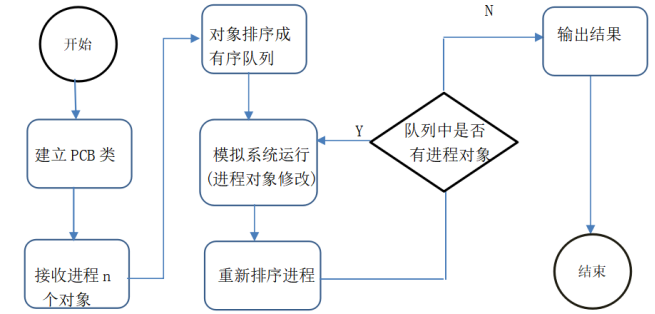五、源程序及注释

#include <iostream>
#include <cstring>
#include <cstdio>
using namespace std;
class PCB                //PCB类，包含time、range、state、next指针
{
public:
void In(char *n,int t,int r);
PCB()
{

}
PCB(PCB *p)                //复制PCB
{
strcpy(name,p->name);
next=p->next;
time=p->time;
range=p->range;
state=p->state;
}
void Red()                    //模拟进程运行状态
{
time--;
range--;
if(!time)
state=0;
}
void print()            //输出当前PCB内容
{
cout<<name<<"\t"<<time<<"\t"<<range<<"\t"<<state<<endl;
}
char name;        //进程名称
PCB *next;            //用于链表的指针
int time;            //用于记录进程运行时间
int range;            //用于记录优先值
int state;            //用于判断该进程是否运行结束
};

void PCB::In(char *n,int t,int r)  //初始化PDB对象
{
strcpy(name,n);
time=t;
range=r;
next=NULL;
if(t>0)
state=1;
else
state=0;
}
{
{
cout<<"进程队列为：";
}
else
cout<<"进程已全部运行完毕。"<<endl;
cout<<endl;
}

void Grp(PCB *&head,PCB * p,int num)//用于队列的排序，num为当前队列中存在的指针个数
{
int ii=num;
if(p->state)            //当该对象为新进程是，插入队列
{
if(!num)
{
}
else
{
for(;num>0;num--)
{
if(p->range > tp->range && ii==num)//位于头部插入
{
break;
}
else if(p->range > tp->range)//位于中间插入
{
p->next=tp;
tp2->next=p;
break;
}
else
{
tp2=tp;
tp=tp->next;
}
}
if(!num && !tp)//位于尾部插入
{
tp2->next=p;
}

}
}
else                    //当该对象是已完成进程时，从队列中除去
{
int tem=num;
for(PCB *tp2=tp;num>0;num--,tp=tp->next)
{
if(!strcmp(p->name,tp->name) && tem==num)
{
break;
}
else if(!strcmp(p->name,tp->name))
{
tp2->next=tp->next;
break;
}
else
tp2=tp;
}

}
}
int Pro(PCB *&p,int sum)   //模拟进程运行，并重新排序队列
{
PCB *k,*pk;
int i;
for(;sum;)
{
k=p;
p->Red();
cout<<"此次运行了进程"<<p->name<<"剩余时间"<<p->time<<"权值"<<p->range<<endl;
if(!k->state)
{
Grp(p,k,sum);
sum--;
}
else if(sum==1)
continue;
else
{
pk=k=k->next;
for(i=sum-1;i>0;i--)
{
if(p->range>=k->range && i==sum-1)//ok
{
break;
}
else if(p->range>=k->range)
{
pk->next=p;
p=p->next;
pk->next->next=k;
break;
}
pk=k;
k=k->next;
}
if(!i && !k)
{
pk->next=p;
p=p->next;
pk->next->next=NULL;
}

}
Pri_Li(p);
}
}
int main()
{
int num,sum,t,r;
char n;
cout<<"这是一个模拟系统加权进程调度程序"<<endl
<<"请输入总的进程数：";
cin>>sum;
PCB *PP[sum];
for(int i=0;i<sum;i++)
{
PP[i]=new PCB;
cout<<"请输入进程名称、进程运行时间、进程优先数：";
cin>>n;

cin>>t;

cin>>r;
PP[i]->In(n,t,r);
num=i;
}
getchar();
return 0;
}

六、打印的程序运行时初值和运行结果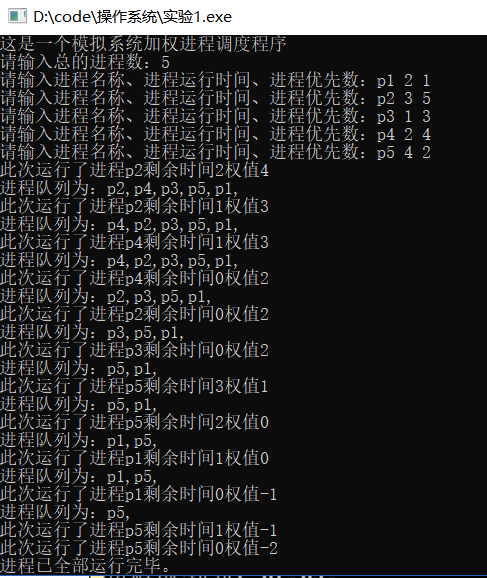七、实验小结

由于理论课的局限性，让我不能很好地理解操作系统的一些算法思路，虽然只是模仿个别思想而做成的这个小程序，但是我从中不但明白了OS程序员们对进程调度的思想，还更好地加深和理解了指针的内层含义，而不是单单地抽象概念。而且C++的程序联系地实在是太少，这同样是一次很好的锻炼。由于不是很顺手，这次试验总共花了我4个多小时，但是其中的乐趣让我忘了午饭同样每一次debug并一步步排除错误后我感到很欣慰，因为我知道，我又成长了。
展开全文• 随机给出一个进程调度实例，如： 进程 到达时间 服务时间 A 0 3 B 2 6 C 4 4 D 6 5 E 8 2 模拟进程调度，给出按照算法先来先服务FCFS、轮转RR（q=1）、最短进程优先SPN、最短剩余时间SRT、最高响应比优先...
• 操作系统实验 处理器调度 时间片轮转 优先数算法
• 实验模拟在单处理器情况下的处理器调度，帮助学生加深了解处理器调度的工作。 三、实验原理 设计一个按优先数调度算法实现处理器调度的程序。 (1) 假定系统有五个进程，每一个进程用一个进程控制块PCB来代表，进程...
• 处理器调度算法: 先来先服务, 时间片轮转, 短作业优先, 最高响应比优先 存储管理: FIFO, LRU 磁盘移臂调度: SSTF, SCAN 文件管理: 运用空闲盘块表的方式组织磁盘空间, 模拟文件的 create() 和 delete() 操作
• 声明 代码虽Low，内容却很实在，思路很清晰，能够实现操作系统处理器调度算法的简单模拟。如果有不对的地方望指正，在下方评论或者私信我均可 ...本实验模拟在单处理器情况下的处理器调度，帮助学...操作系统 Java
• 3、每次运行所设计的处理器调度程序调度进程之前，为每个进程任意确定它的要求运行时间。 4、此程序是模拟处理器调度，因此，被选中的进程并不实际启动运行，而是执行已运行时间+1 来模拟进程的一次运行，表示进程...
• 实验模拟在单处理器情况下的处理器调度，帮助学生加深了解处理器调度的工作。【实验内容】选择一个调度算法，实现处理器调度。【实验指导】本实验有两个题，学生可选择其中的一题做实验。第一题：设计一个按优先数...os
• 实验处理器调度一、实习内容选择一个调度算法，实现处理器调度。；二、实习目的在采用多道程序设计的系统中，往往有若干个进程同时处于就绪状态。当就绪进程个数大于处理器数时，就必须依照某种策略来决定哪些进程...
• 本实习模拟在单处理器环境下的处理器调度，加深了解处理器调度的工作。 三、实习题目 设计一个按时间片轮转法实现处理器调度的程序 [提示]： （1）假定系统有5个进程，每个进程用一个PCB来代表。PCB的结构为： ·...操作系统 C#项目源码 c#
• 随机给出进程调度实例，如 进程 到达时间 服务时间 A 0 3 B 2 6 C 4 4 D 6 5 E 8 2 模拟进程调度，给出按照废先来先服务 FCFS、轮转 RR （q=1）、最短进程优先 SJF、最高响应比优先 HRN、进行调度...C++
• 操作系统，采用先来先服务实现处理器调度，在采用多道程序设计的系统中，往往有若干个进程同时处于就绪状态。当就绪状态进程个数大于...本实验模拟在单处理器情况下处理器调度，帮助学生加深了解处理器调度的工作。
• 操作系统实验，模拟处理器调度 用C/C++实现，按优先数实行调度...